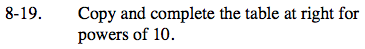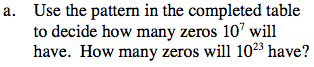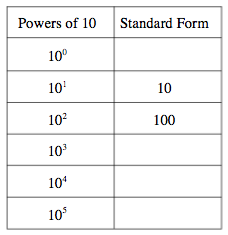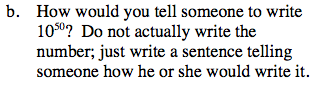Home > ACC7 > Chapter cc38 > Lesson cc38.1.2 > Problem8-19

8-19.
1.  Powers of 10 Standard Form 100 101 10 102 100 103 104 105

Copy and complete the table at right for powers of 10. Homework Help ✎

1. Use the pattern in the completed table to decide how many zeros 107 will have. How many zeros will 1023 have?

2. How would you tell someone to write 1050? Do not actually write the number; just write a sentence telling someone how he or she would write it.Look at 101 = 10 and 102 = 100 closely. What do the exponents tell you about the standard form?

The exponent tells you how many zeros there are in the standard form. Complete the table. How many zeros will 1023 have?Look at the pattern in part (a) and describe how you would find 1050?